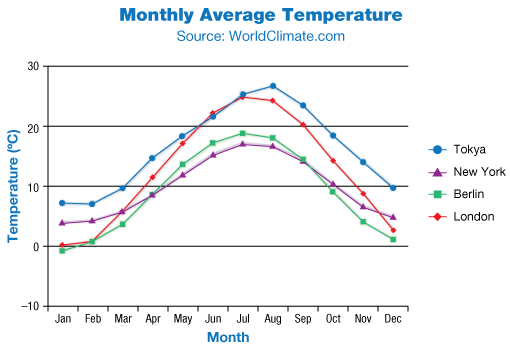# Line Graphs

Go back to  'Data, Statistics'

## Introduction to Line Graphs

Numbers and data keep changing. How do you keep track of data changes and find a way to correlate those changes with time? The answer lies with line graphs. Line graphs have become such an important part of statistical analysis and visualisation that they have moved on from the classroom to becoming crucial topics in quality control, stock price research and predictive analysis.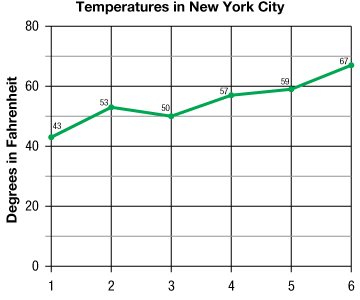## The Big Idea: What Are Line Graphs?

Line graphs are a special kind of data visualisation tool that focuses primarily on time studies. Like other charts, a line chart too has two axes that represent data, but the horizontal axis often represents time or a continuous parameter that tracks the change of the information on the vertical axis.

In comparison, double bar graphs are used to illustrate dual information for one bracket of the categories on the $${x}$$-axis such as the number of boys and girls in each grade. In such a case two charts on the for one grade would indicate both values often with different colours for better separation.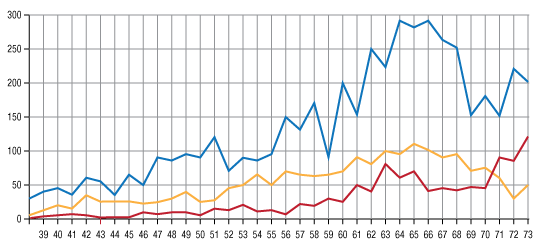Line graphs too have a double chart that can be used to represent two or more data points at the same time bracket as discussed in the next segment.

## Why line graphs? Why are they important?

A line graph requires individual points to be plotted on the two axes and then joined using neighbouring points through straight lines. The vertical axis could represent anything, but the horizontal axis usually represents time.

Line graphs are important for their use in developing continuous trends over time or at least over some quantity that increases sequentially such as the average price of crude oil barrels over thirty years.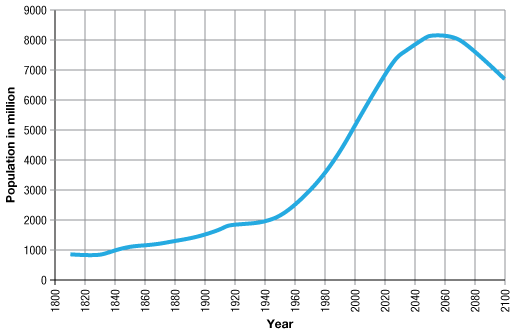The appearance of line graphs is often used to forecast future values using some advanced methods using simple moving averages, exponential moving average and the most commonly used algebraic equations.

The best way to understand these types of graphs can be done on software like Excel. If you simply add some values in two columns and then create a line chart, you can craft a nifty trend equation that allows you to predict future values often regarding $${y}$$ and various degrees of $${x}$$.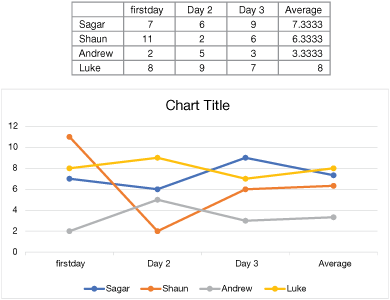Again, it’s important to understand that more accurate trend lines become difficult to produce as the number of data points increase, often creating complex equations of various degrees and orders.

Similarly, a double line graph is a line graph with two lines connecting points to show a continuous change for two objects of interest. Just like a line graph, the lines can drop or rise in a double line graph.

They are used to represent trends in large quantities over time, often to compare and conclude any correlations that may exist between them. Think about the same line chart of the crude oil barrel prices juxtaposed with another line chart for average diesel oil barrel prices.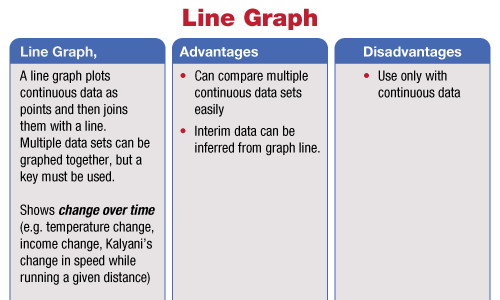Such a chart would help understand the dynamics that one chart has with that of the other.

### Relationship Between Line Graphs and Histograms

The versatility of histograms indicates that they can be used in many different situations. However, it’s important that the data be broken down into specific categories, or at least grouped into categories such that each distinct bar has a specific meaning.

Line charts are in some ways similar to histograms that they represent such data changes over a continuous time frame.

Histograms represent such data regarding data intervals on the horizontal axis but use bars to illustrate them.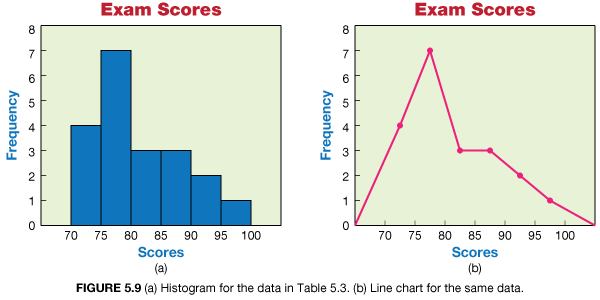The differences between them can be understood through the frequency distributions that they use.

For example, suppose you’re presenting a chart that shows the number of people between all particular age group in a state. The horizontal axis of the histogram would thus have intervals for the age groups in an ascending or descending manner with the vertical axis having the sum of all groups.

Like histograms, trends over time with can be represented using bars with each bar representing each quarter or period. This illustrates a difference. While certain aspects of the line chart can be created from the histogram the same is not possible for the latter.

### When to Use a Line Graph

Bar graphs can show trends over time often to the chagrin of readers and statisticians but suffer many problems for lacking proper ways to represent them.

As a rule of thumb, if the data that is being dealt with has small changes or has any data that relates to time, dates or quarters, use a line graph. They may be less versatile than histograms but can help determine small changes more accurately